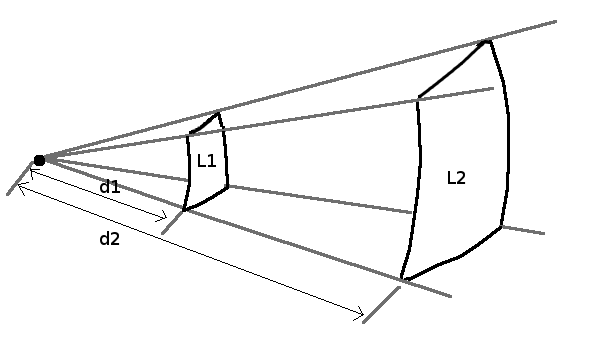# Decibel calculator for sound

This page has a number of different calculators for sound and decibels.

## Add or subtract decibel values

 Add or subtract dB (or dBA) values: result: Available operators: + -

### Use case: Subtract background noise

Measure the sound level in dB or dBA with the sound source running and background noise present. You measure e.g 80dBA. Now turn the sound source off and measure in the same location just the background noise. You measure e.g 75dBA. The background noise has to be more or less constant.
Enter in the calculator 80-75 and press calculate. The result is 78.3dBA
This means the sound source produces 78.3dBA at the point of measurement without background noise.

### Use case: Add sound sources

Measure 3 sound sources separately at the same location (same distance) and then calculate what the resulting dB value would be if they were all running at the same time.
38 + 40 + 29= 42.3dBA

### Formula used by the above calculator

Calculate xdB + ydB:
result=10*Log(10x/10+10y/10) Just extend the formula to add/subtract more values.

## Sound drop over distance, dB over distanceThis calculator uses the Inverse Square Law which allows you to estimate the sound reduction over distance. It can be applied in situations where the sound can propagate freely and is not confined by walls or other large objects. The sound level drops by 6dB when you double the distance to the sound source. 10 times the distance drops the sound level by 20dB. Enter 3 values and the calculator will calculate the 4th value for you. L1: dB at distance d1: m (or ft) L2: dB at distance d2: m (or ft) L = sound level in db or dBA, d = distance in m (meter) or ft (feet). Do not mix units. Use either ft or m.

### Use case: Measure close to the source and calculate the sound level in the distance

Measure the sound level L1 in dB or dBA at the distance d1 from the source. You want to know what the sound level at distance d2 would be.
Example: L1=80dBA at d1=1.5m, What is the sound level at d2=4.5m?
Enter the values 80 (L1), 1.5 (d1) and 4.5 (d2) into the calculator and it will calculate L2=70.5dBA.
Do not get too close to the source when you measure L1 but be close enough such that background noise is neglectable. Common good values are 1.5meter or 2meter for d1.

### Use case: Measure sound at a distant point and calculate the sound level close to the source

Measure the sound level L2 in dB or dBA at the distance d2 from the source. You want to know what the sound level at distance d1 would be.
Example: L2=50dBA at d2=100m, What is the sound level at d1=1.5m?
Enter the values 50 (L2), 100 (d2) and 1.5 (d1) into the calculator and it will calculate L1=86.5dBA.

### Formula used by the above calculator

i2/i1=(d1/d2)2
ΔL=10*Log(i2/i1)
where ΔL is the difference in sound levels (L1-L2) in dB (or dBA).
The quotient i2/i1 is the sound intensity ratio.

This formula works as long as you have spherical wavefronts. Some sound sources such as e.g a large fan may produce parallel wavefronts directly at the fan and they become spherical only as you go further away. d1 must therefore not be too small. Common good values for d1 are 1m (3ft) to 2m (6ft) dependent on the size of the sound emitting object.

## dB to ratio

The unit dB is really just a logarithmic ratio between two numbers. With sound it is as well used as an absolute value and in this case it is calibrated to the lowest sound level that the human ear can hear.

The weakest audible sound is 0 dB and a quiet conversation is 100000 times more intense or 50 dB higher.

Sound level: dB
Result:
```Intensity ratio:
Pressure ratio:
```

Explanations:
Sound intensity: Intensity is the amount of energy transmitted per second over an area. It is measured in watt/m2
Sound pressure: Sound pressure is what microphones measure in the form of the amplitude. It is proportional to the square root of intensity (P=sqrt(I) or I = P2).

## Sound concepts and definitions

• Noise:
Noise is unwanted sound which may be hazardous to health, interfere with speech and verbal communications or is otherwise disturbing, irritating or annoying.

• Sound:
Sound is as any pressure variation in a gas or liquid that can be detected by the human ear.

• Wavelength:
wavelength = velocity/frequency
The velocity or speed of sound in air is 340m/s.

• dB vs dBA:
Humans do not hear all frequencies equally. To account for this, different weightings have been created. "A" weighted sound levels are referred to dBA. See https://en.wikipedia.org/wiki/A-weighting.